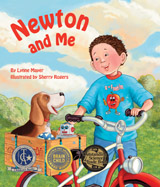Alignment to Standards for TN1 SC-1.11.1. Realize the basic concept that forces can move objects (push/pull).
1 SC-1.11.1.a Observe and describe how the movement of an object can be changed (e.g. push/pull, fast/slow).
2 SC-2.11.1. Realize the basic concept that forces can move objects (push/pull).
3 SC-3.11.1. Realize the basic concept that forces can move objects (push/pull).
3 SC-3.11.1.a Describe the relationship between the amount of force applied to an object and the distance the object moves.
3 SC-3.11.1.b Recognize that objects move differently on different surfaces.
4 SC-4.11.2. Recognize the relationship between force and motion.
4 SC-4.11.2.a Identify factors that affect the amount of friction.
4 SC-4.11.3. Recognize that the motion of objects is affected by friction.
5 SC-5.11.2. Recognize the relationship between force and motion.
5 SC-5.11.2.b Explain how slope affects the amount of force.
5 SC-5.11.3. Recognize that the motion of objects is affected by friction.
5 SC-5.11.3.a Explore and explain how friction affects motion.
K SC-K.11.1. Realize the basic concept that forces can move objects (push/pull).
K SC-K.11.1.a Recognize that objects can move in different directions and at different speeds.
K SC-K.12.1. Recognize that objects have observable properties that can change over time and under different conditions.

Back to Standards Page